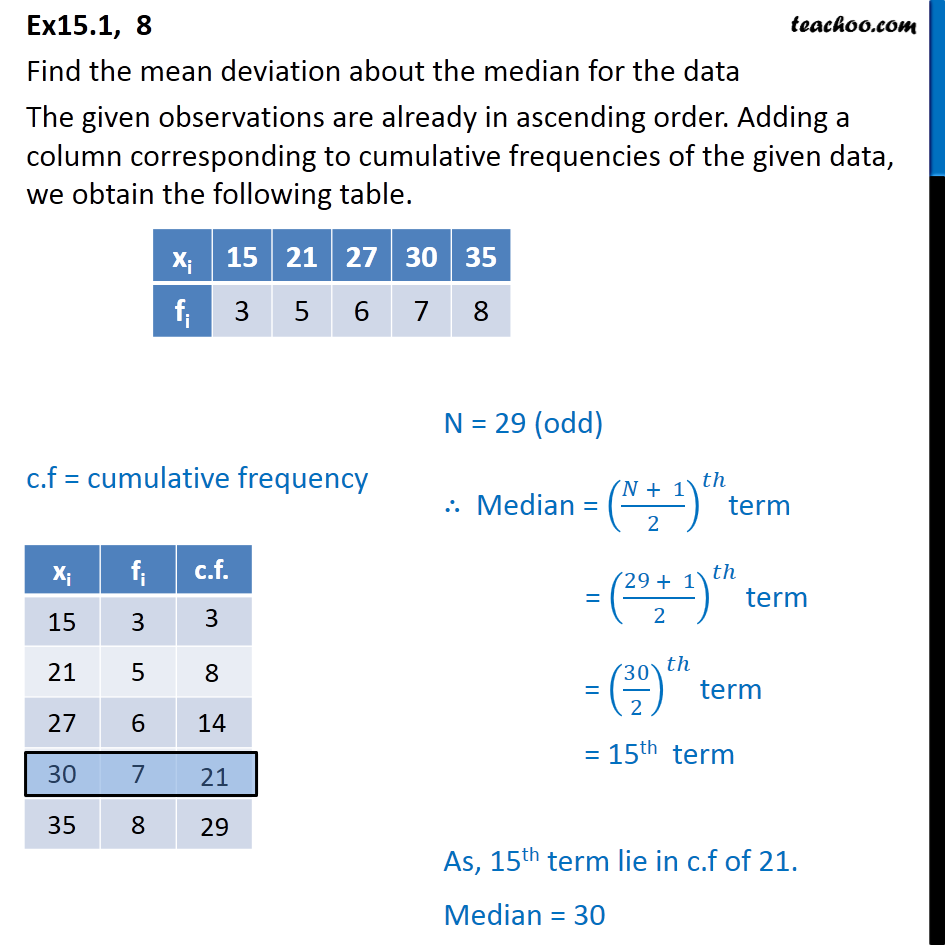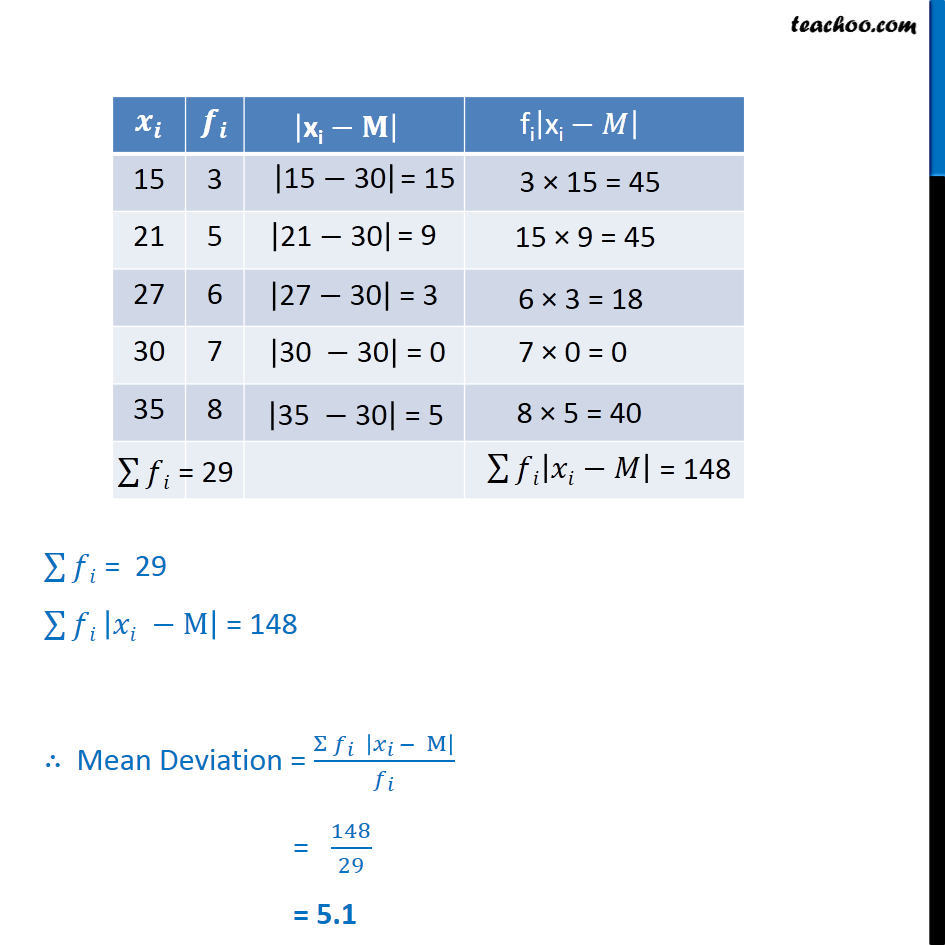Ex 13.1

Chapter 13 Class 11 Statistics
Serial order wiseLearn in your speed, with individual attention - Teachoo Maths 1-on-1 Class

### Transcript

Ex15.1, 8 Find the mean deviation about the median for the data The given observations are already in ascending order. Adding a column corresponding to cumulative frequencies of the given data, we obtain the following table. c.f = cumulative frequency ∑128▒𝑓𝑖 = 29 ∑128▒𝑓𝑖 |𝑥𝑖 −M| = 148 ∴ Mean Deviation = (Σ 𝑓_𝑖 |𝑥_(𝑖 )− M|)/𝑓_𝑖 = 148/29 = 5.1